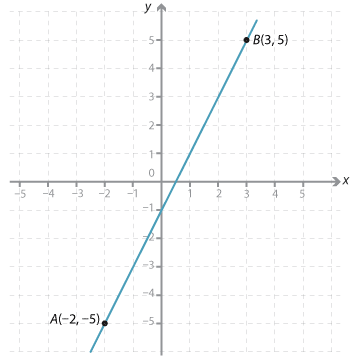### A line in the plane is defined by two points

A line in the plane is defined by two points. We can sketch a line if we know the coordinates of two points on the line and furthermore, if we are given the coordinates of two points on the line, we can find the equation of the line.

#### Example 3

Plot the points (–2, –5) and (3, 5) and draw the straight line through them.

#### SolutionDetailed description

Looking at the graph of the straight line through the two points we have been given, we can see some other points on the graph that the line passes through.

We can place these values in a table together with the two points we were given as shown below.

 x y –2 –1 0 1 2 3 –5 –3 –1 1 3 5

As the x-values increase by one, the y-values increase by two.

The rule is:

• 'Multiply the x-value by 2 and subtract 1'.
• We can write the rule using algebra as y = 2x – 1.

Also, from the equation we can find the coordinates of other points on the line.

For example, when

\begin{align}x = 2, y &= 2 × 2\ – 1\\ &= 3\end{align}

We have done this by inspection. Later, we shall learn to do this systematically.